## Tamilnadu Samacheer Kalvi 10th Maths Solutions Chapter 3 Algebra Ex 3.8

10th Maths Exercise 3.8 Samacheer Kalvi Question 1.
Find the square root of the following polynomials by division method
(i) x4 – 12x3 + 42x2 – 36x + 9
(ii) 37x2 – 28x3 + 4x4 + 42x + 9
(iii) 16x4 + 8x2 + 1
(iv) 121x4 – 198x3 – 183x2 + 216x + 144
Solution:
The long division method in finding the square root of a polynomial is useful when the degrees of a polynomial is higher.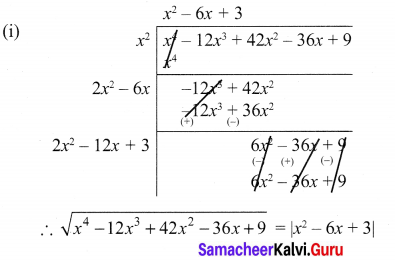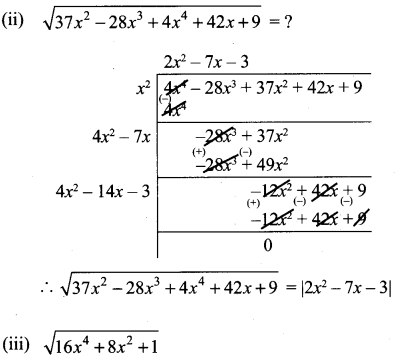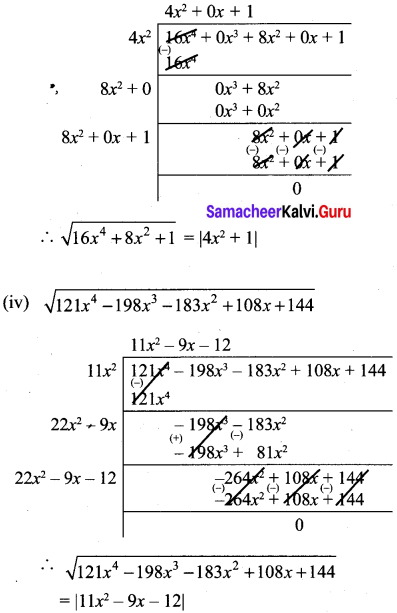Ex 3.8 Class 10 Samacheer Question 2.
Find the square root of the expression $$\frac{x^{2}}{y^{2}}-10 \frac{x}{y}+27-10 \frac{y}{x}+\frac{y^{2}}{x^{2}}$$
Solution: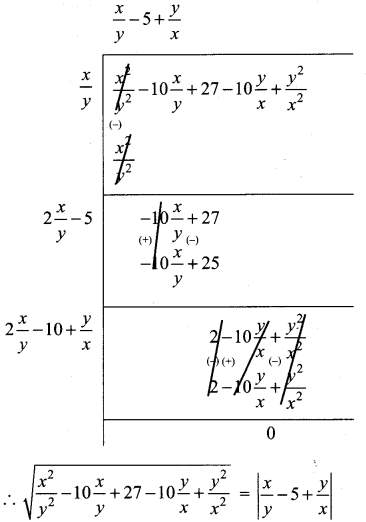10th Maths Exercise 3.8 Question 3.
Find the values of a and b if the following polynomials are perfect squares
(i) 4x4 – 12x3 + 37x2 + bx + a
(ii) ax4 + bx3 + 361ax2 + 220x + 100
Solution:
(i)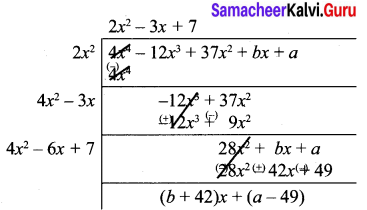Since it is a perfect square.
Remainder = 0
⇒ b + 42 = 0, a – 49 = 0
b = -42, a = 49

(ii) ax4 + bx3 + 361ax2 + 220x + 100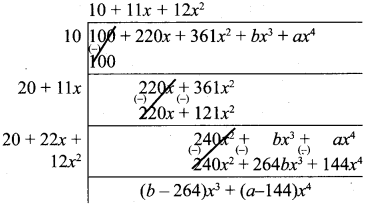Since remainder is 0
a = 144
b = 264

Exercise 3.8 Class 10 Samacheer Question 4.
Find the values of m and n if the following expressions are perfect squares
(i) $$\frac{1}{x^{4}}-\frac{6}{x^{3}}+\frac{13}{x^{2}}+\frac{m}{x}+n$$
(ii) x4 – 8x3 + mx2 + nx + 16
Solution:
(i)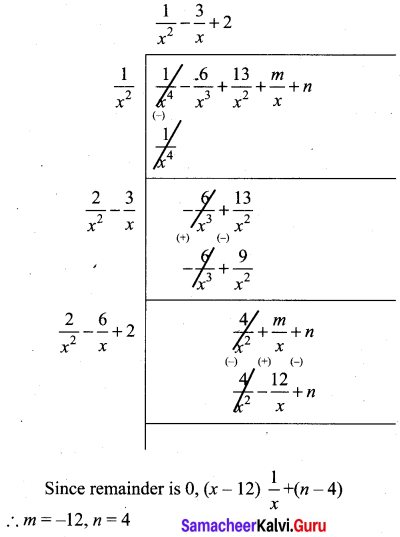(ii)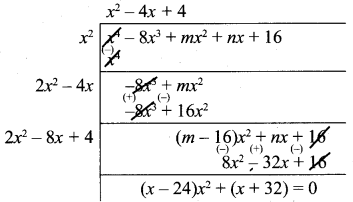Since remainder is 0,
m = 24, n = -32